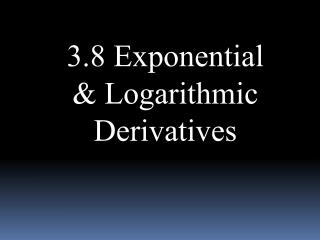DownloadDownload Presentation3.8 Exponential & Logarithmic Derivatives

# 3.8 Exponential & Logarithmic Derivatives

Télécharger la présentation## 3.8 Exponential & Logarithmic Derivatives

- - - - - - - - - - - - - - - - - - - - - - - - - - - E N D - - - - - - - - - - - - - - - - - - - - - - - - - - -
##### Presentation Transcript

1. 3.8 Exponential & Logarithmic Derivatives

2. Exponential Function

3. Log Functions

4. Ex 1: Find y’

5. Ex 2: Evaluate

6. Logarithmic Differentiation: • Take ln of both sides • Use Log Laws to split into separate ln’s • Differentiate Implicitly • Solve for y’

7. Ex 3: Find y’

8. Constant Rule • Power Rule 4 cases:

9. Exponential Function • Function Raised to a Function 4 cases:

10. HW – 3.8 pg. 245#3, 7, 11, 17, 19, 21, 29, 41, 48